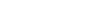# MCP3428 problem with Wire.endTransmission

I have a setup to measure the water level of several water tanks. The hardware is a MCP3428 4-Channel board ( PR 33-15 ) connected to Arduino Uno ( in connection with PR14-3 ) or Arduino Due ( connected with PR37-10 ), to measure the water level with several sensors 4-20mA. Power supply of the sensors is a Mean Well 24 VDC. According to the water depth of 3 to 4 meters, the signal has a current of a max. 8 to 9 mA, which is coherent and corresponds to the scope of the sensors.
The setup has been completed with an isolator ISO1541 and was operational only for a few weeks with correct measurements.
Surprisingly the software now hangs with the command code Wire.endTransmission. Sometimes an error code 2 is transmitted, mostly the program hangs.
The sketch follows the logic of the sample code in “MCP3428_OneShot_Conversion”. This is the sketch:

#include <MCP3428.h>
#include <Wire.h>
unsigned long loop_y = 0;
float raw, volum;
float surface = { 0.2529, 0.2648, 0.3334 };
float water_level, height;
int basis = 2980;
int divisor = 1123;
int resolution = 16;
String text1 = “”;
int delay_var = 5000;
byte error;
int i;
void setup()
*{ *

• Serial.begin(9600);*

• while (!Serial) {;} // wait for serial port to connect.*
}
void loop()
{

• MCP3428 MCP(0); // Declaration of MCP3428: A2, A1, A0 bits (000, 0x68)*

• float volum_total = 0;*

• loop_y++;*

• error = Wire.endTransmission();*

• Serial.println("Wire.endTransmission with error = "+String(error));*

• if (error == 0)*

• ``````  {*
``````
• ``````   for ( i=0; i <= 2; i++)*
``````
• ``````      {*
``````
• ``````        MCP.SetConfiguration(i+1,resolution,1,1);*
``````
• ``````        raw[i] = MCP.readADC();*
``````
• ``````        water_level = raw[i]; *
``````
• ``````        height = (water_level - basis) * 100 / divisor;*
``````
• ``````        volum[i] = height * surface[i];*
``````
• ``````        volum_total = volum_total + volum[i];*
``````
• ``````        text1 = "channel "+String(i+1)+" : resolution "+String(resolution)+", digital measured value: "+String(raw[i],0)+", represents water level: "+String(height, 0)+" cm, corresponds: "+String(volum[i],2)+" cbm";*
``````
• ``````        Serial.println(text1);*
``````
• ``````      }*
``````
• ``````    Serial.println("total volum: "+String(volum_total,2)+" cbm, reading: "+String(loop_y)+" every "+String(delay_var / 1000)+" seconds");*
``````
• ``````    Serial.println("");*
``````
• ``````  }*
``````
• delay(delay_var);*
}

Usually the first Serial.println before Wire.endTransmission is executed, then the sketch hangs. It seems to me, that there is a hardware failure of the MCP3428.
Is there a possibility to reset this device to default settings ? After a few weeks in use, I am hesitant to order a new device. There is actually no more idea to resolve this. Could you please advise me how to find a solution. Thank you.

Wire.EndTransmission is likely waiting for an acknowledgement from the device. The device is not acknowledging, which is causing your code to throw an error. There is a problem communicating on the I2C bus, weather it be a hardware issue is hard to say. I would try to scan the I2C bus to see the start address of all connected devices. This is typically the first step in troubleshooting I2C communications. Unfortunately, I am not a Arduino developer, so I do not have specific instructions on how to do this, but a google search for code should turn up something that will help you see what I2C addresses are properly reporting.